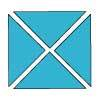#### You may also like### Chocolate

There are three tables in a room with blocks of chocolate on each. Where would be the best place for each child in the class to sit if they came in one at a time?### Four Triangles Puzzle

Cut four triangles from a square as shown in the picture. How many different shapes can you make by fitting the four triangles back together?### Cut it Out

Can you dissect an equilateral triangle into 6 smaller ones? What number of smaller equilateral triangles is it NOT possible to dissect a larger equilateral triangle into?

# Fractions in a Box

##### Age 7 to 11Challenge Level

Remember the booklet in the corner of the box is square, so how many holes could it take up?
The whole box, without the space for the booklet, would fit $100$ discs, so the number of discs must be $100$, less the square number taken up by the booklet.
What do you know about the factors of the total number of discs?